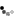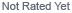Loading... Please wait...

Using a MOSFET to control a DC Motor

Using a MOSFET to control a DC Motor

There is a current limit of 40ma for an Arduino pin. So how can we control circuits that require larger currents such as motors or even mains circuits? There are three key ways of doing this and they each have their own advantages and disadvantages:

1. Relays – mechanical or solid-state. This is the easiest way of controlling mains circuits – where simple on/off control is required. Solid-sate relays are typically used where safe operating conditions preclude mechanical action that may create sparks. Opto-isolators are often used to further isolate the mains circuit from the low-level circuit.
2. Where mains circuits are being varied (for example a light dimmer) then a triac can be used often in conjunction with an opto-isolator. They can be used for on-off switching too.
3. The simplest circuit for motor control is the use of a transistor. It’s possible to use a transistor (BJT or a MOSFET) but the MOSFET applies infinitesimal load on the driving circuit so it has a clear advantage.

Using the supplied MOSFETs to drive a DC motor

Without going into the complexities of the MOSFET we can treat it as a switch. If we apply a potential to the Gate it will switch the current through the Source/Drain junction. So we connect the gate to the Arduino through a 220 ohm current limiting resistor to protect the Arduino. Then we connect the motor to the external power supply and the Drain of the MOSFET. We complete the circuit by connecting up the grounds. In this case the MOSFET controlling the motor is driven from Arduino Pin 9.

CircuitCode

/*

Speed up the motor

This example shows how to control the speed of a DC motor an LED on pin 9 using the analogWrite() function. This example based on the Arduino Example Fade sketch but modified to use timing instead of the delay() function

*/

int turns = 0; // how fast the motor runs

int turnAmount = 1; // how many turns the motor makes

unsigned long currentTime;

unsigned long loopTime;

void setup() {

// declare pin 9 to be an output:

pinMode(9, OUTPUT);

currentTime = millis();

loopTime = currentTime;

}

void loop() {

currentTime = millis();

if(currentTime >= (loopTime + 20)){

// set the speed of pin 9:

analogWrite(9, turns);

// change the turnings for next time through the loop:

turns = turns + turnAmount;

// speed up or slow down the motor

if (turns == 0 || turns == 255) {

turnAmount = -turnAmount ;

}

if (turns == 0) {

delay(5000);

}

loopTime = currentTime; // Updates loopTime

}

// Other processing can be done here

}

New Products

•••••What's News

• Using a Generic STM32 board with Arduino

Using a Generic STM32 Board with ArduinoIf you haven't got one already, you can …

• Using a SIM800l GSM/GPRS Cellphone Module to send an SMS message

This example shows how to use a low-cost SIM800l GSM/GPS Module to send an SMS …

• Using MQTT with ESP8266

This blog entry is quick outline of using MQTT with a message broker to …